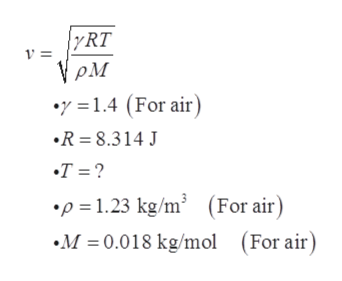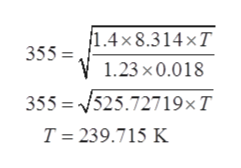# A 33 kHz soundwave travels in air at v=355 m/sWhat is the temperature of the air through which it travels?What is the velocity and wavelength?

Question
16 views

A 33 kHz soundwave travels in air at v=355 m/s

What is the temperature of the air through which it travels?

What is the velocity and wavelength?

check_circle

Step 1

What is the velocity and wavelength?

Given data:

Frequency of the soundwave, f  = 33 kHz = 33000 Hz

Velocity of sound wave in air, v = 355 m/s

Step 2

(a)

The formula:help_outlineImage Transcriptionclose|yRT γρΜ •y =1.4 (For air) •R = 8.314 J •T = ? •p = 1.23 kg/m² (For air) •M = 0.018 kg/mol (For air) fullscreen
Step 3

Plugging in the given values:

...help_outlineImage Transcriptionclose1.4× 8.314 ×T 355 =, 1.23 x 0.018 355 = 525.72719×T T = 239.715 K fullscreen

### Want to see the full answer?

See Solution

#### Want to see this answer and more?

Solutions are written by subject experts who are available 24/7. Questions are typically answered within 1 hour.*

See Solution
*Response times may vary by subject and question.
Tagged in

### Other